# VI. Analysis of Release Catalogs

## 6. Position Reconstruction Performance

### c. Band-Frame Distortion

i. Distortion Checks at 6-Month Intervals:

Using a technique described below, band-frame level distortion has been checked at 6 month intervals for both the Northern and Southern telescopes. The range of nights going into each check point was limited to one week. Due to differences in source density and weather the number of points available varied. Each check point required restoring the necessary pipeline files from several nights of processing and running GenDXY on the Cal scans from each night to generate the needed dxys files of bias-removed USNOA differences. The dxys files for each set of nights were then combined and the distortion was fitted using FitDXY.

Northern telescope model-fit distortion results at the 6 month check points are presented for x-scan_J (Figure 1), in-scan_J (Figure 2), x-scan_H (Figure 3), in-scan_H (Figure 4), x-scan_Ks (Figure 5) and in-scan_Ks (Figure 6). In each figure, mean distortion as per the model fit is plotted as a function of x-scan frame position (xf) in the upper panel and in-scan frame position (yf) in the lower panel. One line is plotted for each of the 6 month check points, coded as follows:

05/97 = black line with "x" symbols

11/97 = red line with open square symbols

05/98 = green line with open triangle symbols

11/98 = blue line with open star symbols

05/99 = magenta line with solid triangle symbols

11/99 = orange line with solid square symbols

05/00 = light grey line with solid star symbols

Southern telescope model-fit distortion results at the 6 month check points are presented for x-scan_J (Figure 7), in-scan_J (Figure 8), x-scan_H (Figure 9), in-scan_H (Figure 10), x-scan_Ks (Figure 11) and in-scan_Ks (Figure 12). In these plots the check points are coded as follows:

03/98 = black line with "x" symbols

09/98 = red line with open square symbols

03/99 = green line with open triangle symbols

09/99 = blue line with open star symbols

03/00 = magenta line with solid triangle symbols

ii. Global Fit- Assuming the Distortion Remains Constant:

The preceding plots have shown very little change in distortion with time for either the Northern or Southern telescopes. Combining the data from all the 6 month check points with data now being produced during pipeline production runs, overall band-frame distortion fits were done for each hemisphere. These fits benefited from very large sample sizes of 2.2, 1.9 and 1.5 million for J, H and Ks, respectively, in the north; and 3.9, 3.4 and 2.3 million for J, H and Ks, respectively, in the south.

Two-dimensional plots for the Northern telescope showing the uncorrected J-band distortion (Figure 13), the model-fit J-band distortion (Figure 14) and the residual J-band distortion (Figure 15) after correction are presented (plotted at 100 times actual size). Corresponding plots for the uncorrected H-band distortion (Figure 16), the model-fit H-band distortion (Figure 17) and the residual H-band distortion (Figure 18) after correction are also presented. Followed by plots for the uncorrected Ks-band distortion (Figure 19), the model-fit Ks-band distortion (Figure 20) and the residual Ks-band distortion (Figure 21). Note that in all three cases the distortion model does a fine job of removing the observed distortion.

The same two-dimensional plots for the Southern telescope showing the uncorrected J-band distortion (Figure 22), the model-fit J-band distortion (Figure 23) and the residual J-band distortion (Figure 24) after correction are presented. Corresponding plots for the uncorrected H-band distortion (Figure 25), the model-fit H-band distortion (Figure 26) and the residual H-band distortion (Figure 27) after correction are also presented. Followed by plots for the uncorrected Ks-band distortion (Figure 28), the model-fit Ks-band distortion (Figure 29) and the residual Ks-band distortion (Figure 30). The distortion model does even better in the south.

iii. Global Fit Residuals at 6-Month Check Points:

It has been demonstrated that the global fits do an excellent job of removing distortion as determined from mean differences taken over the whole data set. How well do the global fits handle each of the 6-month check points? To address this question, two-dimensional plots of mean residuals after the global distortion corrections were generated at each of the check points. The results presented below show that:

1) Compared to the original distortion, the global fit residuals are _small at all check points.

2) Check points with more noticeable global fit residuals typically have fewer measurement points "npts" available.

For the northern j-band, plots for the May 1997 (Figure 31) (npts=169523), Nov 1997 (Figure 32) (npts=327565), May 1998 (Figure 33) (npts=446188), Nov 1998 (Figure 34) (npts=279113), May 1999 (Figure 35) (npts=167882), Nov 1999 (Figure 36) (npts=179375) and May 2000 (Figure 37) (npts=219644) check points are presented.

Northern J-band Check Points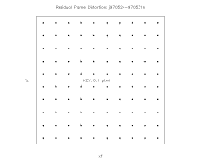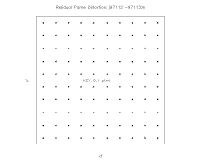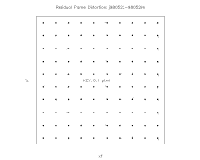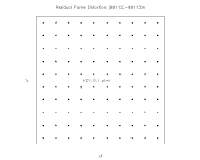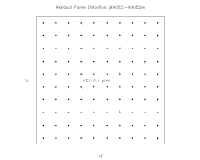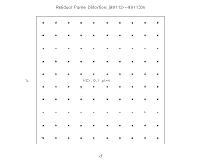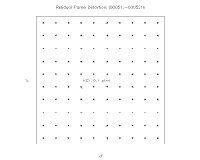Figure 31Figure 32Figure 33Figure 34Figure 35Figure 36Figure 37
May 1997Nov 1997May 1998Nov 1998May 1999Nov 1999May 2000
npts=169523npts=327565npts=446188npts=279113npts=167882npts=179375npts=219644

For the northern h-band, plots for the May 1997 (Figure 38) (npts=148518), Nov 1997 (Figure 39) (npts=274887), May 1998 (Figure 40) (npts=399476), Nov 1998 (Figure 41) (npts=229990), May 1999 (Figure 42) (npts=142100), Nov 1999 (Figure 43) (npts=153987) and May 2000 (Figure 44) (npts=190703) check points are also presented.

Northern H-band Check Points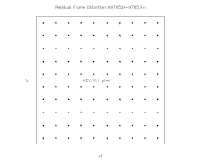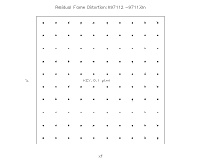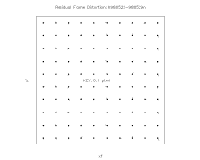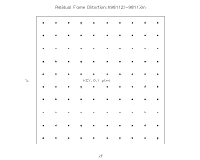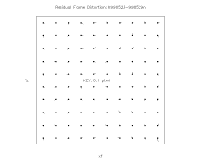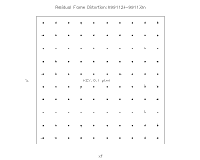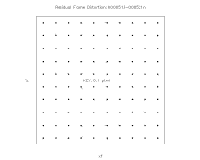Figure 38Figure 39Figure 40Figure 41Figure 42Figure 43Figure 44
May 1997Nov 1997May 1998Nov 1998May 1999Nov 1999May 2000
npts=148518npts=274887npts=399476npts=229990npts=142100npts=153987npts=190703

For the northern k-band, plots for the May 1997 (Figure 45) (npts=104997), Nov 1997 (Figure 46) (npts=194789), May 1998 (Figure 47) (npts=372287), Nov 1998 (Figure 48) (npts=166210), May 1999 (Figure 49) (npts=90899), Nov 1999 (Figure 50) (npts=103297) and May 2000 (Figure 51) (npts=147598) check points are presented as well.

Northern K-band Check Points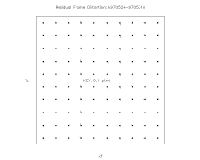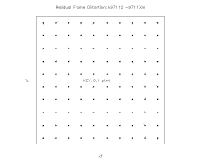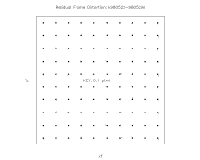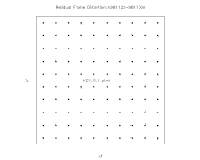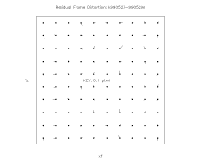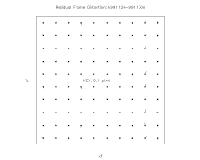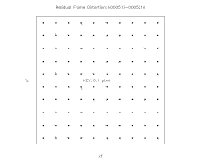Figure 45Figure 46Figure 47Figure 48Figure 49Figure 50Figure 51
May 1997Nov 1997May 1998Nov 1998May 1999Nov 1999May 2000
npts=104997npts=194789npts=372287npts=166210npts=90899npts=103297npts=147598

For the southern j-band, plots for the Mar 1998 (Figure 52) (npts=449011), Sep 1998 (Figure 53) (npts=200980), Mar 1999 (Figure 54) (npts=767384), Sep 1999 (Figure 55) (npts=299924) and Mar 2000 (Figure 56) (npts=759791) check points are presented.

Southern J-band Check Points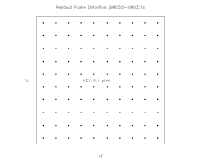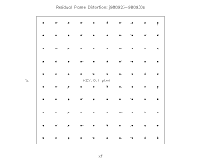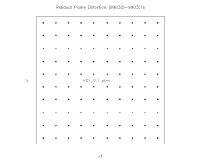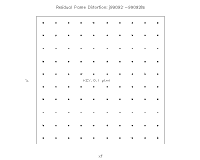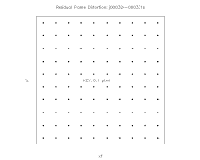Figure 52Figure 53Figure 54Figure 55Figure 56
Mar 1998Sep 1998Mar 1999Sep 1999Mar 2000
npts=449011npts=200980npts=767384npts=299924npts=759791

For the southern h-band, plots for the Mar 1998 (Figure 57) (npts=407909), Sep 1998 (Figure 58) (npts=178710), Mar 1999 (Figure 59) (npts=695391), Sep 1999 (Figure 60) (npts=274099) and Mar 2000 (Figure 61) (npts=689207) check points are also presented.

Southern H-band Check Points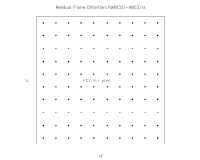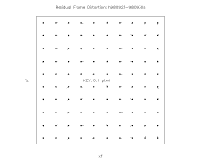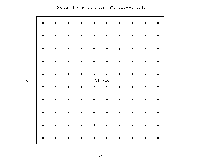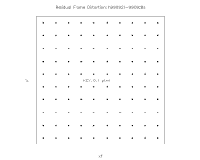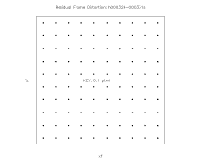Figure 57Figure 58Figure 59Figure 60Figure 61
Mar 1998Sep 1998Mar 1999Sep 1999Mar 2000
npts=407909npts=178710npts=695391npts=274099npts=689207

For the southern k-band, plots for the Mar 1998 (Figure 62) (npts=272569), Sep 1998 (Figure 63) (npts=118956), Mar 1999 (Figure 64) (npts=453939), Sep 1999 (Figure 65) (npts=211580) and Mar 2000 (Figure 66) (npts=468258) check points are presented as well.

Southern K-band Check Points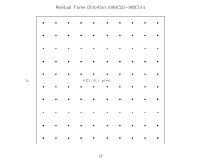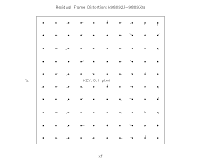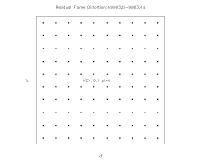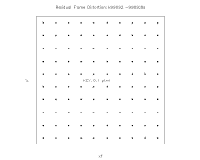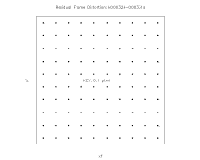Figure 62Figure 63Figure 64Figure 65Figure 66
Mar 1998Sep 1998Mar 1999Sep 1999Mar 2000
npts=272569npts=118956npts=453939npts=211580npts=468258

It appears likely the increased residuals at some check points are due to limitations on the number of available measurements and therefore may not reflect actual changes in the distortion. This is supported by the fact that the model-fit results from Section I (which are less sensitive to measurement counts) show little change between check points.

iv. Computing Distortion Using USNO-A2.0

Up until now distortion has been computed from special scans of Stone's astrometric fields. See descriptions of distortion calculations for the North and South using Stone fields. Keeping in mind that we are only interested in relative positions for the distortion analysis, it should be possible to use an inherently lower accuracy catalog such as the USNO-A2.0 to determine the distortions. Provided pertinent biases can be removed, the increased standard deviation of the USNO-A2.0 can be compensated for by using more points. Being able to use the USNO-A2.0 to compute distortion has some definite advantages. Since all calibration scans are reconstructed using the USNO-A2.0, there is no need to re-run PosFrm with a new set of reference stars before beginning the analysis. Any sufficiently large set of calibration scans can be used as input to the distortion analysis.

As a test of concept, I took the calibration scans from the night of 981005s, which happened to be on line, as input to the distortion analysis. After any remaining biases were removed a band at a time, the USNO-A2.0 sources used as reference stars by PosFrm were mapped into individual band-frame coordinates. These were matched to 2MASS extractions with high quality positions and position differences were computed. After some trimming, the x-scan (dx) and in-scan (dy) differences were fitted separately for each band to the following polynomial:

del =c1*x^2 +c2*y*x^2 +c3*x*y +c4*x*y^2 +c5*y^2 +c6*x +c7*y +c8 +c9*x^3 +c10*y^3

Figure 67 plots the average x-scan distortion in J-band as a function of x-scan frame position in the upper-left panel and as a function of in-scan frame position position in the lower-left panel. Note that the units are pixels. The in-scan distortion is plotted in the two panels to the right. The same presentation is made for H-band in Figure 68 and for K-band in Figure 69. In each plot the solid black lines refer to the measured distortion distortion and the dotted red lines to the polynomial fit.

The average difference values from these plots show more variation than seen in the Stone South distortion analysis. The modeling, however, smooths out those variations and ends up quite close to the Stone results. This is illustrated by Figure 70, which compares J-band model distortion using the USNO-A2.0 (dotted red line) to that obtained using the Stone field (black line). The comparison is repeated for H-band in Figure 71 and K-band in Figure 72.

The matches are not bad and should get even better if more USNO-A2.0 differences were used. Given that the scatter from a USNO-A2.0 fit is about three times as large as that from a Stone fit, one would expect to need three squared (9) times as many sources to get the same model quality. Using all the calibrations from the night of 981005s falls far short of that criteria:

```
Stone Count    USNO-A2.0 Count   Ratio USN/Stone

J-band    15528           23591            1.52
H-band    14826           20208            1.36
K-band    14422           12259            0.85

```
K-band USNO-A2.0 counts are the most deficient and correspondingly show the largest model differences. The fact that the USNO-A2.0 fit did as well as it did probably reflects the fact that there was considerable overkill in the Stone counts. In any case, we should be able to use more than one night's calibration scans to get the counts up.

In conclusion, it appears that quality distortion analysis can be done using USNO-A2.0 residuals from pipeline processing of the calibration scans. In fact, it would be good to add to the PosFrm script the capability to call a program which computes the frame level differences needed for a distortion analysis and saves those differences to an historical file. That would allow us to determine if (and how) distortion varies with time by selecting appropriate subsets of that file as inputs to the distortion analysis.

That said, I still feel that it would be prudent to scan additional Stone fields from time to time as a truth test.

v. Northern Distortion as Determined from 980113n/Stone (Field e)

Herein are figures presenting distortion for all three bands as determined from 8 scans of Stone's deep/dense field "e" on 980113n. First, scans 057 through 064 were reconstructed using the Stone sources in addition to ACT sources as reference stars. Approximately 2 degrees of each scan overlapped the Stone field. After any residual scan bias was removed, the Stone source positions were mapped into individual frame coordinates and differences w.r.t. 2MASS extractions computed. The x-scan (dx) and in-scan (dy) differences were fitted separately for each band to the following polynomial:

del =c1*x^2 +c2*y*x^2 +c3*x*y +c4*x*y^2 +c5*y^2 +c6*x +c7*y +c8 +c9*x^3 +c10*y^3

Figure 73 plots the average x-scan distortion in J-band as a function of x-scan frame position in the upper-left panel and as a function of in-scan frame position in the lower-left panel. Note that the units are pixels. The in-scan distortion is plotted in the two panels to the right. The same presentation is made for H-band in Figure 74 and for K-band in Figure 75. In each plot the solid black lines refer to the measured distortion and the dotted red lines to the polynomial fit. Note that the averaged fits track the average observed differences very well in all cases.

The distortion fit reduces the standard deviation of the residuals between 3 and 14 percent, depending on the band and direction. The improvement is illustrated by before/after histograms shown in Figure 76 for J-band, Figure 77 for H-band, and Figure 78 for K-band. As before, the solid black lines are without the fit and the dotted red lines are with the fit. When limited to Read1-Read2 extractions only, the improvement is even greater and varies from 5 to 22 percent.

My thanks to Gene Kopan for a set of plots showing a two-dimensional representation of the measured distortion. Figure 79 breaks the J-band field up into a 10x10 grid and plots arrows showing the direction and magnitude of the distortion in each of the 100 grid squares. The vector is plotted at 100 times its actual length. Figure 80 does the same for H-band and Figure 81 repeats for K-band.

The polynominal distortion model has been coded and delivered with ver 2.0 of PosMan, but is currently disabled via namelist flag until it can be incorporated in ProPhot. This analysis should be repeated in the future to see if there's any significant change with time or telescope orientation.

vi. Southern Distortion as Determined from 980801s/Stone (Field m)

Herein are figures presenting distortion with the Southern instrument for all three bands a s determined from 24 scans of Stone's deep/dense field "m" on 980801s. First, scans 010-021 and 068-079 were reconstructed using the Stone sources as reference stars. Although each scan was only approximately one degree long, the field is dense and all 24 scans are totally contained therein. After any remaining biases were removed a band at a time, the Stone source positions were mapped into individual band-frame coordinates. These were matched to 2MASS extractions with high quality positions and position differences were computed. The x-scan (dx) and in-scan (dy) differences were fitted separately for each band to the following polynomial:

del =c1*x^2 +c2*y*x^2 +c3*x*y +c4*x*y^2 +c5*y^2 +c6*x +c7*y +c8 +c9*x^3 +c10*y^3

Figure 82 plots the average x-scan distortion in J-band as a function of x-scan frame position in the upper-left panel and as a function of in-scan frame position in the lower-left panel. Note that the units are pixels. The in-scan distortion is plotted in the two panels to the right. The same presentation is made for H-band in Figure 83 and for K-band, which has the most distortion, in Figure 84. In each plot the solid black lines refer to the measured distortion and the dotted red lines to the polynomial fit. Note that the averaged fits track the average observed differences very well in all cases.

The improvement with the distortion model, although largely swamped by larger random errors, can be seen in the before/after histograms shown in Figure 85 for J-band, Figure 86 for H-band, and Figure 87 for K-band. As before, the solid black lines are without the fit and the dotted red lines are the fit. As expected, the K-band improvements stand out.

The next set of plots show a two-dimensional representation of the measured distortion. Figure 88 breaks the J-band field up into a 10x10 grid and plots arrows showing the direction and magnitude of the distortion in each of the 100 grid squares. The vector is plotted at 100 times its actual length. Figure 89 does the same for H-band and Figure 90 repeats for K-band. In all three plots a 0.1 pixel long horizonal measuring stick is presented at plot center. Note that for K-band the worst-case distortions an the corners approach 0.2 pixels. Although worst-case distortions of ~0.2 pixels were also seen in the North, fewer of the 100 grid squares had such large values.

[Last Updated: 2004 Sep 21; by H. McCallon and R. Tam]

Previous page. Next page.